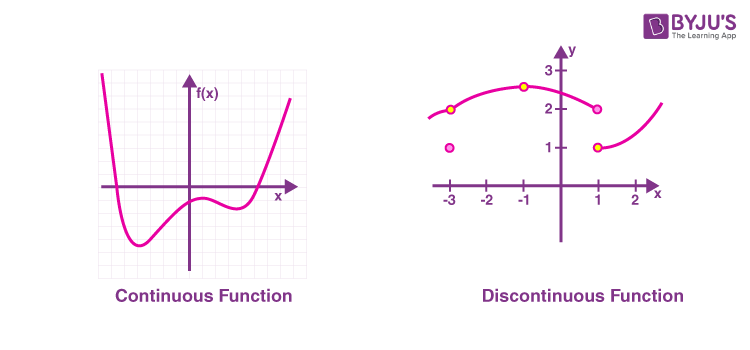Know more about the Repeaters Batch Know more about the Repeaters Batch

# Continuity Of A Function

Continuity of a function is an important concept in differential calculus. Questions are frequently asked in competitive exams and JEE exams from this topic. In this article, we discuss the concept of Continuity of a function, condition for continuity, and the properties of continuous function. We can say that a function is continuous, if we can plot the graph of a function without lifting our pen. If we lift our pen to plot a certain part of a graph, we can say that it is a discontinuous function.

The following graph shows a continuous and discontinuous function.## Condition For Continuity

Mathematically, a function f(x) is said to be continuous at a point x = a, iff

• f(a) exists
• $$\begin{array}{l}\lim_{x\to a}f(x)=f(a)\end{array}$$
• $$\begin{array}{l}\lim_{x\to a^{-}}f(x)=\lim_{x\to a^{+}}f(x)=f(a)\end{array}$$
. i.e LHL = RHL = f(a)

If any one of the above conditions is not satisfied, then the function is said to be discontinuous at a.

Consider the following example.

$$\begin{array}{l}f(x)=\begin{Bmatrix} 5-2x\: for\: x<1\\ 3\: for \: x=1& \\x+2\: for\: x>1& \end{Bmatrix}\end{array}$$

Check whether the function is continuous for all x.

Solution:

Let us check the conditions for continuity.

Given f(1) = 3

LHL:

$$\begin{array}{l}\lim_{x\to 1^{-}}f(x)=5-2=3\end{array}$$

RHL:

$$\begin{array}{l}\lim_{x\to 1^{+}}f(x)=x+2=1+2=3\end{array}$$

LHL = RHL. Also f(1) exists.

So the function is continuous at x = 1.

Hence f(x) is continuous for all x.

### Properties of Continuous Functions

Consider two functions f(x) and g(x), both continuous at x = a. Then

1. f(x) + g(x) will be continuous at x = a

2. f(x) – g(x) will be continuous at x = a.

3. c.f(x) will be continuous at x = a, where c is any constant

4. f(x).g(x) will be continuous at x = a.

5. f(x)/g(x) is continuous at x = a, (g(a) ≠ 0).

Limits Continuity And Differentiability

### Solved Examples

Example 1:

If f(x) is continuous and f(9/2) = 2/9, then limx→0 f(1-cos3x)/x2 is equal to

a) 9/2

b) 0

c) 2/9

d) 8/9

Solution:

Given f(9/2) = 2/9

limx→0 f(1-cos3x)/x2 = f (limx→0 (1-cos3x)/x2)

Use L’hospital rule

= f (limx→03 sin 3x/2x)

Use L’hospital rule

= f (limx→0 9 cos 3x/2)

= f(9/2)

= 2/9

Hence option c is the answer.

Example 2:

If f(x) = 1/x – (k-1)/(e2x-1), x ≠0, is continuous at x = 0, then the ordered pair (k, f(0)) equal

a) (⅓, 2)

b) (3, 2)

c) (2, 1)

d) (3, 1)

Solution:

For the function to be continuous at x = 0, limx→0 f(x) exists and is equal to f(0).

Given f(x) = (1/x) – (k-1)/(e2x-1)

limx→ 0f(x) = limx→ 0 (1/x) – (k-1)/(e2x-1)

= limx→ 0 {(e2x-1 – kx + x)}/x(e2x-1)

[e2x = 1+2x+(2x)2/2! + (2x)3/3! +….]

limx→ 0 {(e2x-1 – kx + x)}/x(e2x-1) = limx→ 0 [(1+2x+(2x)2/2! + (2x)3/3! +..)-1-k+x]/[(x(1+2x+(2x)2/2! + (2x)3/3! +…)-1)]

= limx→ 0 [(3-k)x + (4x2/2!) + 8x3/3! +…]/[2x2 + 4x3/2! + 8x4/3! + …]

For the limit to exist, the power of x in the numerator should be greater than or equal to the power of x in the denominator.

So the coefficient of x in the numerator is equal to zero.

3-k = 0

=> k = 3

The limit reduces to limx→ 0 (x2)(4/2! + 8x/3! + …)/x2(2+ 4x/2! + 8x2/3! +…)

= limx–>0 (4/2! + 8x/3! +…)/(2 + 4x/2! + 8x2/3!+…)

= 4/4

= 1

So f(0) = 1

The ordered pair (k, f(0)) = (3, 1)

Hence option d is the answer.

Related video### How do you know if a function is continuous?

We can use the following condition to check whether a function is continuous or not. A function f(x) continuous at a point x = a, if f(a) exists, lim x→a f(x) = f(a) and lim x→ a- f(x) = lim x→ a+ f(x) = f(a). (LHL = RHL).

### List two properties of Continuous Functions.

Let f(x) and g(x) be two functions which are continuous at x = a. The product of the functions is continuous.

f(x).g(x) will be continuous at x = a.
f(x)/g(x) is continuous at x = a, (g(a) ≠ 0).

### What do you mean by Discontinuous function?

A function which is not continuous is called a discontinuous function. The graph of a discontinuous function will break somewhere.